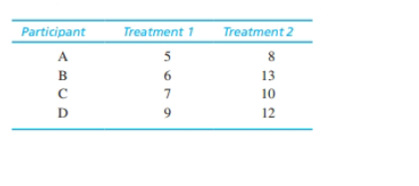Chapter 11.2, Problem 1LCEssentials of Statistics for the B...

8th Edition
Frederick J Gravetter + 1 other
ISBN: 9781133956570

Solutions

Chapter
SectionEssentials of Statistics for the B...

8th Edition
Frederick J Gravetter + 1 other
ISBN: 9781133956570
Textbook Problem

For the following data from a repeated-measures study, find the difference scores and compute the sample mean difference, the variance for the difference scores, and the standard error for the sample mean difference.To determine
The sample mean difference, the variance for the difference score and standard errors for the sample mean difference for the given data.

Explanation

Given info:

The given data are shown below,

 Participants Treatment 1 Treatment 2 A 5 8 B 6 13 C 7 10 D 9 12

Calculation:

The difference and D of the data set is obtained below:

 Participants Treatment 1 Treatment 2 D D2 A 5 8 3 9 B 6 13 7 49 C 7 10 3 9 D 9 12 3 9 TOTAL 16 76

The value of MD is,

MD=DN=164=4

The value of MD is 4

Still sussing out bartleby?

Check out a sample textbook solution.

See a sample solution

The Solution to Your Study Problems

Bartleby provides explanations to thousands of textbook problems written by our experts, many with advanced degrees!

Get Started

Find the numerical value of each expression. 1. (a) sinh 0 (b) cosh 0

Single Variable Calculus: Early Transcendentals, Volume I

Solve the equations in Exercises 112 for x (mentally, if possible). x+1=3x+1

Finite Mathematics and Applied Calculus (MindTap Course List)

Given the Taylor Series , a Taylor series for ex/2 is:

Study Guide for Stewart's Single Variable Calculus: Early Transcendentals, 8th

0 1 ∞

Study Guide for Stewart's Multivariable Calculus, 8th